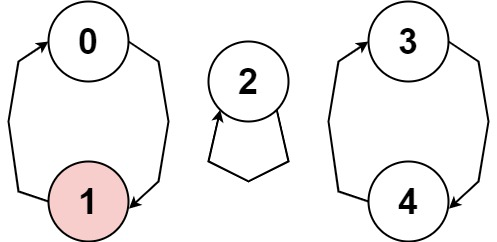## Algorithm

Problem Name: 457. Circular Array Loop

You are playing a game involving a circular array of non-zero integers `nums`. Each `nums[i]` denotes the number of indices forward/backward you must move if you are located at index `i`:

• If `nums[i]` is positive, move `nums[i]` steps forward, and
• If `nums[i]` is negative, move `nums[i]` steps backward.

Since the array is circular, you may assume that moving forward from the last element puts you on the first element, and moving backwards from the first element puts you on the last element.

A cycle in the array consists of a sequence of indices `seq` of length `k` where:

• Following the movement rules above results in the repeating index sequence `seq -> seq -> ... -> seq[k - 1] -> seq -> ...`
• Every `nums[seq[j]]` is either all positive or all negative.
• `k > 1`

Return `true` if there is a cycle in `nums`, or `false` otherwise.

Example 1:```Input: nums = [2,-1,1,2,2]
Output: true
Explanation: The graph shows how the indices are connected. White nodes are jumping forward, while red is jumping backward.
We can see the cycle 0 --> 2 --> 3 --> 0 --> ..., and all of its nodes are white (jumping in the same direction).
```

Example 2:```Input: nums = [-1,-2,-3,-4,-5,6]
Output: false
Explanation: The graph shows how the indices are connected. White nodes are jumping forward, while red is jumping backward.
The only cycle is of size 1, so we return false.
```

Example 3:```Input: nums = [1,-1,5,1,4]
Output: true
Explanation: The graph shows how the indices are connected. White nodes are jumping forward, while red is jumping backward.
We can see the cycle 0 --> 1 --> 0 --> ..., and while it is of size > 1, it has a node jumping forward and a node jumping backward, so it is not a cycle.
We can see the cycle 3 --> 4 --> 3 --> ..., and all of its nodes are white (jumping in the same direction).
```

Constraints:

• `1 <= nums.length <= 5000`
• `-1000 <= nums[i] <= 1000`
• `nums[i] != 0`

## Code Examples

### #1 Code Example with Javascript Programming

```Code - Javascript Programming```

``````
const circularArrayLoop = function(nums) {
let n = nums.length;
for (let i = 0; i < n; i++) {
if (nums[i] == 0) {
continue;
}
let j = i,
k = getNextIndex(i, nums);
while (nums[k] * nums[i] > 0 && nums[getNextIndex(k, nums)] * nums[i] > 0) {
if (j === k) {
// check for loop with only one element
if (j === getNextIndex(j, nums)) {
break;
}
return true;
}
j = getNextIndex(j, nums);
k = getNextIndex(getNextIndex(k, nums), nums);
}
// loop not found, set all element along the way to 0
j = i;
let val = nums[i];
while (nums[j] * val > 0) {
let next = getNextIndex(j, nums);
nums[j] = 0;
j = next;
}
}
return false;
};

function getNextIndex(i, nums) {
const n = nums.length;
return i + nums[i] >= 0 ? (i + nums[i]) % n : n + ((i + nums[i]) % n);
}
``````
Copy The Code &

Input

cmd
nums = [2,-1,1,2,2]

Output

cmd
true